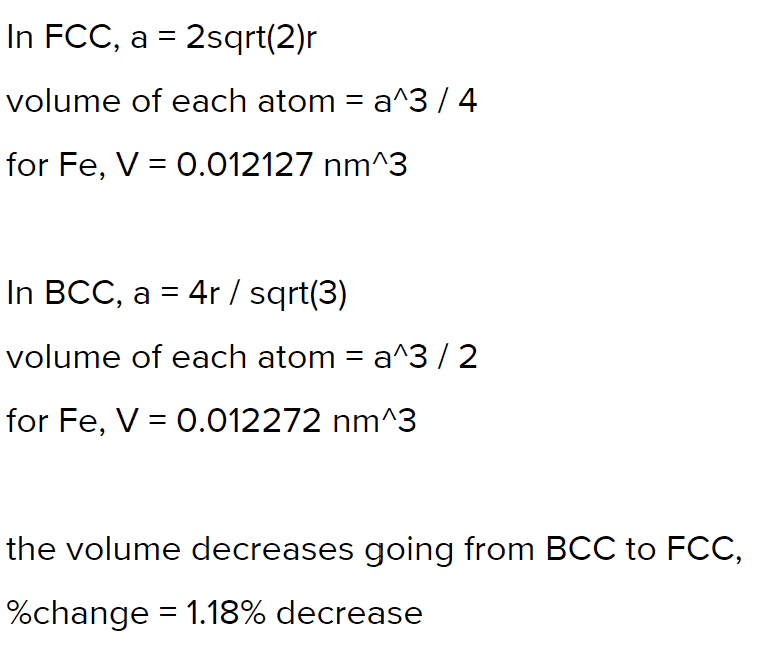# Should the # of atoms in a unit cell be considered to find the % volume change?

• Engineering
catlip
Homework Statement:
Iron (Fe) undergoes an allotropic transformation at 912°C: upon heating from a BCC (α phase) to an FCC (γ phase). Accompanying this transformation is a change in the atomic radius of Fe—from RBCC = 0.12584 nm to RFCC = 0.12894 nm—and, in addition, a change in density (and volume). Compute the percentage volume change associated with this reaction. Indicate a decreasing volume by a negative number.
Relevant Equations:
V=a^3
First of all, I don't think the question was clear enough. Therefore, I had to assume they are referring to the volume of the unit cell.
V=a^3, side length a
aBCC=2R√2, aFCC=4√3/3R
%change=(VFCC-VBCC)/VBCC
I thought this was right until I checked with others who did this:so the only difference is including the number of atoms per unit cell (4 and 2). But wouldn't this actually be the %change in density?